# Select labels using machine learning#

apoc allows selecting labeled objects according to properties such as size, shape and intensity in a corresponding image. In this example, we will select elongated objects from an instance segmentation of blobs.

import apoc

import pyclesperanto_prototype as cle
import numpy as np

cle.select_device('RTX')

<NVIDIA GeForce RTX 3050 Ti Laptop GPU on Platform: NVIDIA CUDA (1 refs)>

image = imread('../../data/blobs.tif')
labels = cle.label(cle.threshold_otsu(image))

cle.imshow(image)
cle.imshow(labels, labels=True)
cle.imshow(annotation, labels=True)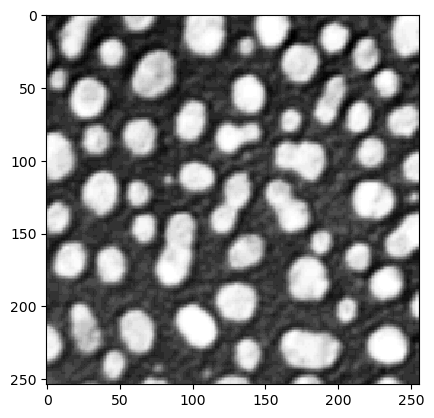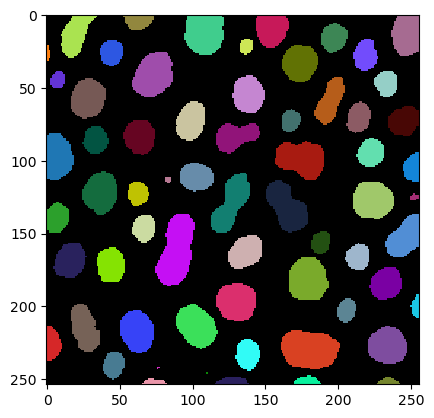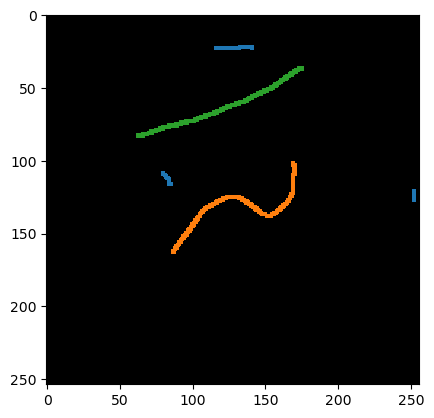## Training#

For training the classifier, you need to specify features. In the following we use mean and standard deviation intensity within the labeled objects and the object size and shape.

features = 'area,mean_max_distance_to_centroid_ratio,standard_deviation_intensity'

cl_filename = "object_selector.cl"

# Create an object classifier
apoc.erase_classifier(cl_filename) # delete it if it was existing before
classifier = apoc.ObjectSelector(cl_filename, positive_class_identifier=1)

# train it
classifier.train(features, labels, annotation, image)


## Prediction#

After the classifier was trained, we can use it to select objects.

result = classifier.predict(labels, image)

print(result.max())

cle.imshow(result, labels=True)

23.0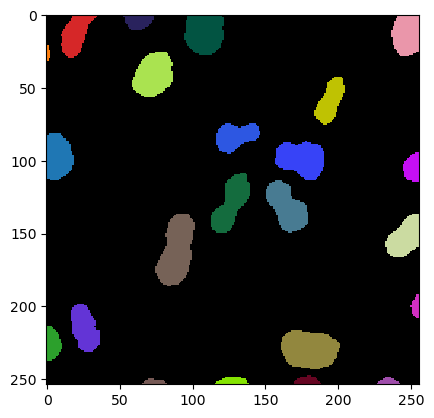One can also load the classifier from disc and apply it to another dataset. We demonstrate that by applying the classifier to a rotated version of the image and label image from above.

image1 = image.T
labels1 = cle.label(cle.threshold_otsu(image1))

classifier = apoc.ObjectSelector(cl_filename)

result = classifier.predict(labels1, image1)

print(result.max())

cle.imshow(result, labels=True)

23.0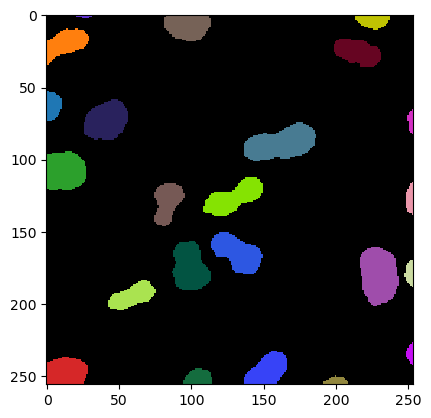After training, we can ask the classifier how important features were while doing the prediction.

classifier.feature_importances()

{'area': 0.29573084473661354,
'mean_max_distance_to_centroid_ratio': 0.4264564597125618,
'standard_deviation_intensity': 0.27781269555082466}


# Exercise#

Use the code and example images above to train a classifier that selects all small objects in the label image.